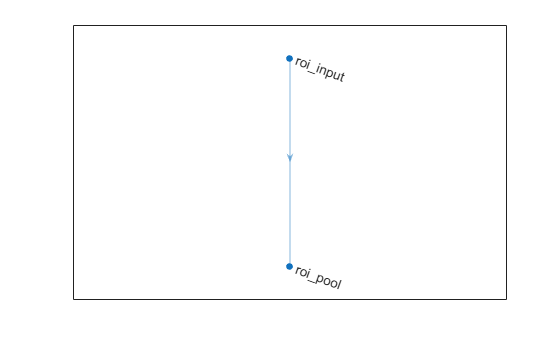# roiMaxPooling2dLayer

Neural network layer used to output fixed-size feature maps for rectangular ROIs

## Description

An ROI max pooling layer outputs fixed size feature maps for every rectangular ROI within the input feature map. Use this layer to create a Fast or Faster R-CNN object detection network.

Given an input feature map of size [H W C N], where C is the number of channels and N is the number of observations, the output feature map size is [height width C `sum`(M)], where height and width are the output size. M is a vector of length N and M(i) is the number of ROIs associated with the i-th input feature map.

There are two inputs to this layer:

• `'in'` — The input feature map that will be cropped

• `'roi'` — A list of ROIs to pool

Use the input names when connecting or disconnecting the ROI max pooling layer to other layers using `connectLayers` (Deep Learning Toolbox) or `disconnectLayers` (Deep Learning Toolbox) (requires Deep Learning Toolbox™).

## Creation

### Syntax

``layer = roiMaxPooling2dLayer(outputSize)``
``layer = roiMaxPooling2dLayer(outputSize,'Name',Name)``

### Description

````layer = roiMaxPooling2dLayer(outputSize)` creates a max pooling layer for ROIs and sets the `OutputSize` property.```

example

````layer = roiMaxPooling2dLayer(outputSize,'Name',Name)` creates a max pooling layer for ROIs and sets the optional `Name` property. To create a network containing an ROI max pooling layer, you must specify a layer name.```

## Properties

expand all

Pooled output size, specified as a two-element vector of positive integers of the form ```[height width]```.

Layer name, specified as a character vector or a string scalar. For `Layer` array input, the `trainNetwork`, `assembleNetwork`, `layerGraph`, and `dlnetwork` functions automatically assign names to layers with `Name` set to `''`.

Data Types: `char` | `string`

Number of inputs of the layer. This layer has two inputs.

Data Types: `double`

Input names of the layer. This layer has two inputs, named `'in'` and `'roi'`.

Data Types: `cell`

Number of outputs of the layer. This layer has a single output only.

Data Types: `double`

Output names of the layer. This layer has a single output only.

Data Types: `cell`

## Examples

collapse all

Create an ROI input layer.

`roiInput = roiInputLayer('Name','roi_input');`

Create an ROI max pooling layer with output size [4 4].

```outputSize = [4 4]; roiPool = roiMaxPooling2dLayer(outputSize,'Name','roi_pool');```

Add the layers to a LayerGraph.

```lgraph = layerGraph; lgraph = addLayers(lgraph,roiInput); lgraph = addLayers(lgraph,roiPool);```

Specify that the output of the ROI input layer is the `'roi'` input of the ROI max pooling layer.

```lgraph = connectLayers(lgraph,'roi_input','roi_pool/roi'); plot(lgraph)```| | | (Deep Learning Toolbox) | (Deep Learning Toolbox) | (Deep Learning Toolbox) | (Deep Learning Toolbox)

### Topics

Introduced in R2018b